Download Presentation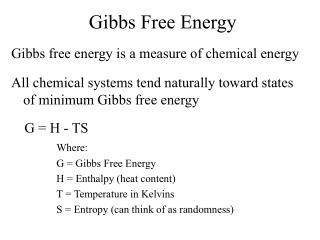Gibbs Free Energy

# Gibbs Free Energy - PowerPoint PPT Presentation

Gibbs Free Energy. Gibbs free energy is a measure of chemical energy. All chemical systems tend naturally toward states of minimum Gibbs free energy. G = H - TS Where: G = Gibbs Free Energy H = Enthalpy (heat content) T = Temperature in Kelvins S = Entropy (can think of as randomness).I am the owner, or an agent authorized to act on behalf of the owner, of the copyrighted work described.
Download Presentation## Gibbs Free Energy

An Image/Link below is provided (as is) to download presentation

Download Policy: Content on the Website is provided to you AS IS for your information and personal use and may not be sold / licensed / shared on other websites without getting consent from its author.While downloading, if for some reason you are not able to download a presentation, the publisher may have deleted the file from their server.

- - - - - - - - - - - - - - - - - - - - - - - - - - E N D - - - - - - - - - - - - - - - - - - - - - - - - - -
Presentation Transcript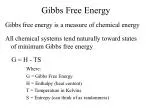Gibbs Free Energy

Gibbs free energy is a measure of chemical energy

All chemical systems tend naturally toward states of minimum Gibbs free energy

G = H - TS

Where:

G = Gibbs Free Energy

H = Enthalpy (heat content)

T = Temperature in Kelvins

S = Entropy (can think of as randomness)Gibbs Free Energy

• Products and reactants are in equilibrium when their Gibbsfree energies are equal
• A chemical reaction will proceed in the direction of lower Gibbs free energy (i.e., DGr < 0)…so the reaction won’t proceed if the reaction produces
• an increase in Gibbs free energy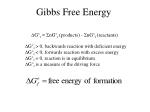Gibbs Free Energy

DG°r = SnG°f (products) - SnG°f (reactants)

DG°r > 0, backwards reaction with deficient energy

DG°r < 0, forwards reaction with excess energy

DG°r = 0, reaction is in equilibrium

DG°r is a measure of the driving force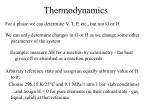Thermodynamics

We can only determine changes in G or H as we change some other parameters of the system

For a phase we can determine V, T, P, etc., but not G or H

Example: measure DH for a reaction by calorimetry - the heat given off or absorbed as a reaction proceeds

Arbitrary reference state and assign an equally arbitrary value of H to it:

Choose 298.15 K/25°C and 0.1 MPa/1 atm/1 bar (lab conditions)

...and assign H = 0 for pure elements (in their natural state - gas, liquid, solid) at that reference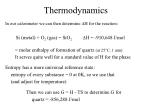Thermodynamics

In our calorimeter we can then determine DH for the reaction:

Si (metal) + O2 (gas) = SiO2 DH = -910,648 J/mol

= molar enthalpy of formation of quartz (at 25°C, 1 atm)

It serves quite well for a standard value of H for the phase

Entropy has a more universal reference state:

entropy of every substance = 0 at 0K, so we use that

(and adjust for temperature)

Then we can use G = H - TS to determine G for

quartz = -856,288 J/mol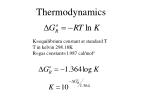Thermodynamics

K=equilibrium constant at standard T

T in kelvin 298.18K

R=gas constant=1.987 cal/molo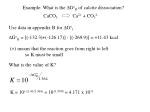Example: What is the DGoR of calcite dissociation?

CaCO3 Ca2+ + CO32-

Use data in appendix B for DGof

DGoR = [(-132.3)+(-126.17)] - [(-269.9)] = +11.43 kcal

(+) means that the reaction goes from right to left

so K must be small

What is the value of K?

K = 10(-11.43/1.364) = 10-8.3798 = 4.171 x 10-9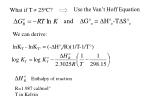Enthalpy of reaction

R=1.987 cal/mol°

T in Kelvin

Use the Van’t Hoff Equation

What if T  25oC?

and

DG°r = DH°r-TDS°r

We can derive:

lnKT - lnKT° = (-DH°r/R)(1/T-1/T°)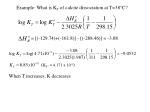= [(-129.74)+(-161.8)] - [(-288.46)] = -3.08

Example: What is KT of calcite dissociation at T=38°C?

(KT° = 4.171 x 10-9)

When T increases, K decreases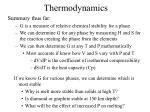Thermodynamics

Summary thus far:

• G is a measure of relative chemical stability for a phase
• We can determine G for any phase by measuring H and S for the reaction creating the phase from the elements
• We can then determine G at any T and P mathematically
• Most accurate if know how V and S vary with P and T
• dV/dP is the coefficient of isothermal compressibility
• dS/dT is the heat capacity (Cp)

If we know G for various phases, we can determine which is most stable

• Why is melt more stable than solids at high T?
• Is diamond or graphite stable at 150 km depth?
• What will be the effect of increased P on melting?